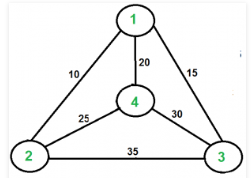2 views

Suppose I have 10 points. I know the distance between each point.

I need to find the shortest possible route passing through all points.

I have tried a couple of algorithms (Dijkstra, Floyd Warshall,...) and they all give me the shortest path between start and end, but they don't make a route with all points on it.

Permutations work fine, but they are too resource-expensive.

What algorithms can you advise me to look into for this problem? Or is there a documented way to do this with the above-mentioned algorithms?

by (108k points)
edited by

Suppose I have 10 points. I know the distance between each point.

I need to find the shortest possible route passing through all points.

I have tried a couple of algorithms (Dijkstra, Floyd Warshall,...) and they all give me the shortest path between start and end, but they don't make a route with all points on it.

Permutations work fine, but they are too resource-expensive.

What algorithms can you advise me to look into for this problem? Or is there a documented way to do this with the above-mentioned algorithms?

Try applying the Travelling Salesman Problem algorithm as it can resolve your query.For example, consider the graph shown in the figure on the right side.

A TSP tour in the graph is 1-2-4-3-1.

The cost of the tour is 10+25+30+15 which is 80.

For n number of vertices or points in a graph, there are (n - 1)! the number of possibilities.

Here is a detailed algorithm about the working of TSP:

C ({1}, 1) = 0 // length between 1 and 1 is 0

for s = 2 to n do // s contains all the points

for all subsets S Є {1, 2, 3, … , n} of size s and containing 1

C (S, 1) = ∞ // length between s and 1 cannot be determined

for all j Є S and j ≠ 1 // j should belong to s and it should not be at the first position

C (S, j) = min {C (S – {j}, i) + d(i, j)// d(i,j) is the distance between i and j points

for i Є S and i ≠ j

}

Return minj C({1, 2, 3, …, n}, j) + d(j, i) // returns the shortest path

Watch this video to learn about Neural Networks:

Go through this Artificial Intelligence Course to get a clear understanding of Artificial Intelligence!